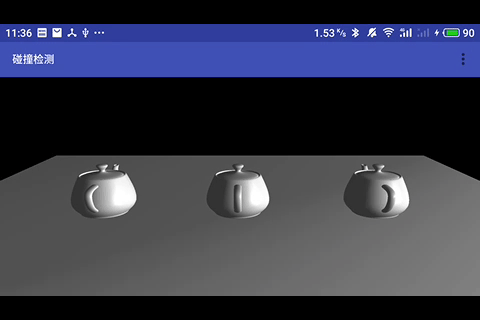# 《OpenGL ES 3.x 游戏开发》碰撞检测之 AABB 包围盒

## 碰撞检测实现原理

AABB 包围盒就是采用一个长方体将物体包裹起来，进行两个物体的相交性检测时仅检测物体对应包围盒（包裹物体的长方体）的相交性。$$x_{min} \le x \le x_{max}$$ $$y_{min} \le y \le y_{max}$$ $$z_{min} \le z \le z_{max}$$

$$P_{min} = [x_{min} , y_{min} , z_{min}]$$ $$P_{max} = [x_{max} , y_{max} , z_{max}]$$

$$c=(P_{min} + P_{max}) / 2$$

## AABB 包围盒计算

public class Vector3f {
float x;//三维变量中的x值
float y;//三维变量中的y值
float z;//三维变量中的z值
public Vector3f(float x,float y,float z)
{
this.x=x;
this.y=y;
this.z=z;
}
{
this.x+=temp.x;
this.y+=temp.y;
this.z+=temp.z;
}
}


public class AABBBox {
float minX;//x轴最小位置
float maxX;//x轴最大位置
float minY;//y轴最小位置
float maxY;//y轴最大位置
float minZ;//z轴最小位置
float maxZ;//z轴最大位置

public AABBBox(float[] vertices)
{
init();
findMinAndMax(vertices);
}

public AABBBox(float minX,float maxX,float minY,float maxY,float minZ,float maxZ)
{
this.minX=minX;
this.maxX=maxX;
this.minY=minY;
this.maxY=maxY;
this.minZ=minZ;
this.maxZ=maxZ;
}
//初始化包围盒的最小以及最大顶点坐标
public void init()
{
minX=Float.POSITIVE_INFINITY;
maxX=Float.NEGATIVE_INFINITY;
minY=Float.POSITIVE_INFINITY;
maxY=Float.NEGATIVE_INFINITY;
minZ=Float.POSITIVE_INFINITY;
maxZ=Float.NEGATIVE_INFINITY;
}
//获取包围盒的实际最小以及最大顶点坐标
public void findMinAndMax(float[] vertices)
{
for(int i=0;i<vertices.length/3;i++)
{
//判断X轴的最小和最大位置
if(vertices[i*3]<minX)
{
minX=vertices[i*3];
}
if(vertices[i*3]>maxX)
{
maxX=vertices[i*3];
}
//判断Y轴的最小和最大位置
if(vertices[i*3+1]<minY)
{
minY=vertices[i*3+1];
}
if(vertices[i*3+1]>maxY)
{
maxY=vertices[i*3+1];
}
//判断Z轴的最小和最大位置
if(vertices[i*3+2]<minZ)
{
minZ=vertices[i*3+2];
}
if(vertices[i*3+2]>maxZ)
{
maxZ=vertices[i*3+2];
}
}
}
//获得物体平移后的AABB包围盒
public AABBBox getCurrAABBBox(Vector3f currPosition)
{
AABBBox result=new AABBBox
(
this.minX+currPosition.x,
this.maxX+currPosition.x,
this.minY+currPosition.y,
this.maxY+currPosition.y,
this.minZ+currPosition.z,
this.maxZ+currPosition.z
);
return result;
}
}


## AABB 包围盒的碰撞检测public boolean check(RigidBody ra, RigidBody rb)//true为撞上
{
float[] over = calOverTotal
(
// 两个物体的 AABB 包围盒
ra.collObject.getCurrAABBBox(ra.currLocation),
rb.collObject.getCurrAABBBox(rb.currLocation)
);
// 三个方向的交叠值与设定的阈值进行比较
return over > V_UNIT && over > V_UNIT && over > V_UNIT;
}

// 传入两个物体的 AABB 包围盒
public float[] calOverTotal(AABBBox a, AABBBox b) {
float xOver = calOverOne(a.maxX, a.minX, b.maxX, b.minX);
float yOver = calOverOne(a.maxY, a.minY, b.maxY, b.minY);
float zOver = calOverOne(a.maxZ, a.minZ, b.maxZ, b.minZ);
return new float[]{xOver, yOver, zOver};
}
// 计算每个轴方向的交叠值
public float calOverOne(float amax, float amin, float bmax, float bmin) {
float minMax = 0;
float maxMin = 0;
if (amax < bmax)//a物体在b物体左侧
{
minMax = amax;
maxMin = bmin;
} else //a物体在b物体右侧
{
minMax = bmax;
maxMin = amin;
}

if (minMax > maxMin) {
return minMax - maxMin;
} else {
return 0;
}
}


## 实践RigidBody 代码如下：

public class RigidBody {
CollisionObject renderObject;//渲染者
AABBBox collObject;//碰撞者
boolean isStatic;//是否静止的标志位
Vector3f currLocation;//位置三维变量
Vector3f currV;//速度三维变量
final float V_UNIT = 0.02f;//阈值

public RigidBody(CollisionObject renderObject, boolean isStatic, Vector3f currLocation, Vector3f currV) {
this.renderObject = renderObject;
collObject = new AABBBox(renderObject.vertices);
this.isStatic = isStatic;
this.currLocation = currLocation;
this.currV = currV;
}

public void drawSelf() {
MatrixState.pushMatrix();//保护现场
MatrixState.translate(currLocation.x, currLocation.y, currLocation.z);
renderObject.drawSelf();//绘制物体
MatrixState.popMatrix();//恢复现场
}

public void go(ArrayList<RigidBody> al) {
if (isStatic) return;
for (int i = 0; i < al.size(); i++) {

RigidBody rb = al.get(i);
if (rb != this) {
if (check(this, rb))//检验碰撞
{
this.currV.x = -this.currV.x;//哪个方向的有速度，该方向上的速度置反
}
}
}
}
// 省略碰撞检测的代码，在上面已经有了。
}


    Vector3f currLocation;//位置三维变量
Vector3f currV;//速度三维变量
// 位置向量加上速度向量，就改变了物体的位置
// translate 方法让改变生效
MatrixState.translate(currLocation.x, currLocation.y, currLocation.z);


                if (check(this, rb))//检验碰撞，如果发生碰撞了，改变方向
{
this.currV.x = -this.currV.x;//哪个方向的有速度，该方向上的速度置反
}


    var aList = ArrayList<RigidBody>() // 定义刚体集合
// onSurfaceCreated 方法里面添加要绘制的物体，
override fun onSurfaceCreated(gl: GL10?, config: EGLConfig?) {
aList.add(RigidBody(ch, true, Vector3f(-13f, 0f, 0f), Vector3f(0f, 0f, 0f)))
aList.add(RigidBody(ch, true, Vector3f(13f, 0f, 0f), Vector3f(0f, 0f, 0f)))
aList.add(RigidBody(ch, false, Vector3f(0f, 0f, 0f), Vector3f(0.1f, 0f, 0f)))
// 开启一个线程来改变刚体的移动
lgt.start()
}
// 绘制刚体
override fun onDrawFrame(gl: GL10?) {
GLES30.glClear(GLES30.GL_DEPTH_BUFFER_BIT or GLES30.GL_COLOR_BUFFER_BIT)
for (it in 0 until aList.size) {
aList[it].drawSelf()
}
pm.drawSelf()
}


public class LovoGoThread extends Thread {
ArrayList<RigidBody> al;//控制列表
boolean flag = true;//线程控制标志位
this.al = al;
}
public void run() {
while (flag) {
int size = al.size();
for (int i = 0; i < size; i++) {
al.get(i).go(al);
}
try {
sleep(20);
} catch (Exception e) {
e.printStackTrace();
}
}
}
}


https://github.com/glumes/AndroidOpenGLTutorial

## 注意点

AABB 包围盒的碰撞检测其实还是一种间接检测方案，所以肯定还是会有误差的。

AABB 包围盒对于本身横平竖直的物体在平行于坐标轴摆放的情况下下，计算碰撞检测的误差很小，但对于不规则形状的物体或本身横平竖直的物体在随意倾斜摆放时，计算碰撞检测的误差就会比较大，如下图所示：## 参考

1. 《OpenGL ES 3.x 游戏开发》

「真诚赞赏，手留余香」#### 欢迎扫码关注微信公众号：【音视频开发进阶】，获得最新文章推送~~~